Left- vs. Right-Handed Coordinate Systems
[ contents ]
Left- vs. Right-handed coordinate systems

#### Left-handed coordinate system

The default coordinate system in the RenderMan(TM) Interface is left-handed: the positive x, y and z axes point right, up and forward, respectively. Positive rotation is clockwise about the axis of rotation.

#### Right-handed coordinate system

The default coordinate system in OpenGL(TM) is right-handed: the positive x and y axes point right and up, and the negative z axis points forward. Positive rotation is counterclockwise about the axis of rotation.positive x rotation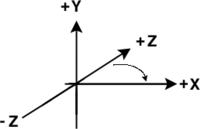positive y rotation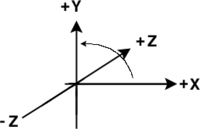positive z rotation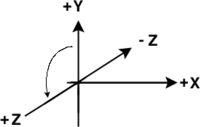positive x rotation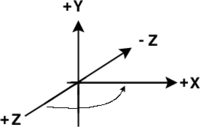positive y rotation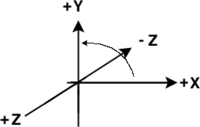positive z rotation